International
Tables for
Crystallography
Volume B
Reciprocal space
Edited by U. Shmueli

International Tables for Crystallography (2010). Vol. B, ch. 2.5, pp. 385-386

## Section 2.5.7.10. Resolution estimation and analysis of errors in single-particle reconstruction

P. A. Penczekg

#### 2.5.7.10. Resolution estimation and analysis of errors in single-particle reconstruction

| top | pdf |

The development of resolution measures in EM was greatly influenced by earlier work in X-ray crystallography. In EM, the problem is somewhat more difficult as, unlike in crystallography, both the amplitude and the phase information in the data are affected by alignment procedures (which we consider distant analogues of phase-extension methods in crystallography). Therefore, resolution measures in EM reflect the self-consistency of the results; however, as the data are subject to alignment, there is a significant risk of introducing artifacts resulting from the alignment of the noise component in the data. Ultimately, these artifacts will unduly improve' the resolution of the map.

The resolution measures used in EM fall into two categories: measures based on averaging of Fourier transforms of individual images and measures based on comparisons of averages calculated for subsets of the data. In the first group, we have the Q-factor (van Heel & Hollenberg, 1980; Kessel et al., 1985) and the spectral signal-to-noise ratio (SSNR) introduced for the 2D case by Unser and co-workers (Unser et al., 1987), and for the 3D case for a class of reconstruction algorithms data are based on direct Fourier inversion by Penczek (Penczek, 2002). The second group of measures includes the differential phase residual (DPR) (Frank et al., 1981) and the Fourier ring correlation (FRC) (Saxton & Baumeister, 1982). A marked advantage of these measures is that they are equally well applicable to 2D or 3D data. In the latter case, the volumes resulting from 3D reconstruction algorithms take the place of the 2D averages.

The resolution measures used in single-particle reconstruction are designed to evaluate the SSNR in the reconstruction as a function of spatial frequency (Penczek, 2002). The resolution' of the reconstruction is reported as a spatial frequency limit beyond which the SSNR drops below a selected level, for example below one.

The FSC is evaluated by taking advantage of the large number of single-particle images: the total data set is randomly split into halves; for each subset a 3D reconstruction is calculated (in two dimensions, a simple average); and two maps f and g are compared in Fourier space,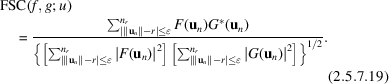In (2.5.7.19), 2is a preselected ring/shell thickness, the un form a uniform grid in Fourier space,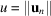is the magnitude of the spatial frequency and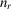is the number of Fourier voxels in the shell corresponding to frequency u. The FSC yields a 1D curve of correlation coefficients as a function of u. Note that the FSC is insensitive to linear transformations of the densities of the objects. An FSC curve everywhere close to one reflects strong similarity between f and g; an FSC curve with values close to zero indicates the lack of similarity between f and g. Particularly convenient for the interpretation of the results in terms of resolution' is the relation between the FSC and the SSNR, which is easily derived by taking the expectation of (2.5.7.19)under the assumption that both f and g are sums of the same signal and different realizations of the noise, which are uncorrelated with the signal and between them (Saxton, 1978):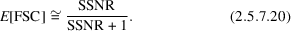By solving (2.5.7.20)for SSNR we obtain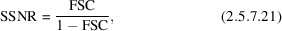which, taking into account that the FSC was calculated from the data set split into halves, has to be modified to (Unser et al., 1987)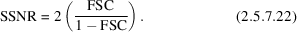In order to calculate the FSC that corresponds to a given SSNR, one inverts (2.5.7.22)to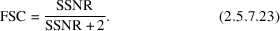Equations (2.5.7.21)and (2.5.7.22)serve as a basis for various resolution criteria' used in EM. The often-used 3σ criterion (van Heel, 1987b) equates resolution with the point at which the FSC is larger than zero at a 3σ level, where σ is the expected standard deviation of the FSC that has an expected value of zero, in essence finding a frequency for which the SSNR is significantly larger than zero. The 3σ criterion has a distinct disadvantage of reporting the resolution at a frequency at which there is no significant signal, while tempting the user to interpret the detail in the map at this resolution. Moreover, as the FSC approaches zero, its relative error increases, so the curve oscillates widely around the zero level increasing the chance of selecting an incorrect resolution point. In other criteria one tries to equate the resolution with the frequency at which noise begins to dominate the signal. A good choice of the cut-off level is SSNR = 1.0, a level at which the power of the signal in the reconstruction is equal to the power of the noise. According to (2.5.7.22), this corresponds to FSC = 0.333. Another often-used cut-off level is FSC = 0.5, at which the SSNR in the reconstruction is 2.0 (Böttcher et al., 1997; Conway et al., 1997; Penczek, 1998).

The main reason behind the determination of the resolution of the EM maps is the necessary step of low-pass filtration of the results before the interpretation of the map is attempted. In order to avoid mistakes, particularly the danger of overinterpretation, one has to remove from the map unreliable Fourier coefficients. Inclusion of Fourier coefficients with a low SNR will result in the creation of spurious details and artifacts in the map. Thus, the optimal filtration should be based on the SSNR distribution in the map and the solution is given by a Wiener filter: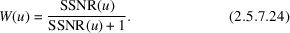Based on the relation of FSC to SSNR (2.5.7.22), we can write (2.5.7.24)as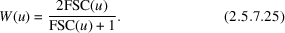In practice, because of the irregular shape of typical FSC curves (particularly for small values of FSC) it is preferable to approximate the shape of the Wiener filter (2.5.7.25)by one of the standard low-pass filters, such as Butterworth (Gonzalez & Woods, 2002) or hyperbolic tangent (Basokur, 1998).

The FRC/FSC methodology can be used to compare a noise-corrupted map with a noise-free ideal version of the same object. In single-particle reconstruction this situation emerges when an X-ray crystallographic structure of either the entire EM-determined structure or of some of its domains is available (Penczek et al., 1999). In this case, we assume that in (2.5.7.19)f represents a sum of the signal and additive uncorrelated noise and g represents the noise-free signal, so is straightforward to calculate the expectation of (2.5.7.19)in order to obtain the relation between the cross-resolution (CRC) and the SSNR: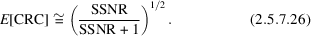Thus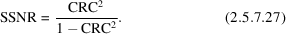Interestingly, for the same SSNR cut-off levels, corresponding values of CRC are higher than those for FSC. For example, for SSNR = 1, CRC = 0.71, while FSC = 0.33. For SSNR = 2, CRC = 0.82, while FSC = 0.5.

### References

Basokur, A. T. (1998). Digital filter design using the hyperbolic tangent functions. J. Balkan Geophys. Soc. 1, 14–18.
Böttcher, B., Wynne, S. A. & Crowther, R. A. (1997). Determination of the fold of the core protein of hepatitis B virus by electron cryomicroscopy. Nature (London), 386, 88–91.
Conway, J. F., Cheng, N., Zlotnick, A., Wingfield, P. T., Stahl, S. J. & Steven, A. C. (1997). Visualization of a 4-helix bundle in the hepatitis B virus capsid by cryo-electron microscopy. Nature (London), 386, 91–94.
Frank, J., Verschoor, A. & Boublik, M. (1981). Computer averaging of electron micrographs of 40S ribosomal subunits. Science, 214, 1353–1355.
Gonzalez, R. F. & Woods, R. E. (2002). Digital Image Processing. Upper Saddle River: Prentice Hall.
Heel, M. van (1987b). Similarity measures between images. Ultramicroscopy, 21, 95–100.
Heel, M. van & Hollenberg, J. (1980). The stretching of distorted images of two-dimensional crystals. In Electron Microscopy at Molecular Dimensions, edited by W. Baumeister, pp. 256–260. Berlin: Springer.
Kessel, M., Radermacher, M. & Frank, J. (1985). The structure of the stalk surface layer of a brine pond microorganism: correlation averaging applied to a double layered lattice structure. J. Microsc. 139, 63–74.
Penczek, P. (1998). Measures of resolution using Fourier shell correlation. J. Mol. Biol. 280, 115–116.
Penczek, P., Ban, N., Grassucci, R. A., Agrawal, R. K. & Frank, J. (1999). Haloarcula marismortui 50S subunit – complementarity of electron microscopy and X-ray crystallographic information. J. Struct. Biol. 128, 44–50.
Penczek, P. A. (2002). Three-dimensional spectral signal-to-noise ratio for a class of reconstruction algorithms. J. Struct. Biol. 138, 34–46.
Saxton, W. O. (1978). Computer Techniques for Image Processing of Electron Microscopy. New York: Academic Press.
Saxton, W. O. & Baumeister, W. (1982). The correlation averaging of a regularly arranged bacterial envelope protein. J. Microsc. 127, 127–138.
Unser, M., Trus, B. L. & Steven, A. C. (1987). A new resolution criterion based on spectral signal-to-noise ratios. Ultramicroscopy, 23, 39–51.